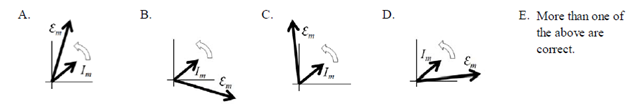# Problem: A driven RLC circuit is set up such that at its resonant frequency R = X C = XL. If the driving frequency is instead twice the resonant frequency, which the following is the phasor diagram for current and emf voltage? Consider Im to be at 45° in all options.

###### FREE Expert Solution
89% (154 ratings)
###### Problem Details

A driven RLC circuit is set up such that at its resonant frequency R = X C = XL. If the driving frequency is instead twice the resonant frequency, which the following is the phasor diagram for current and emf voltage? Consider Im to be at 45° in all options.